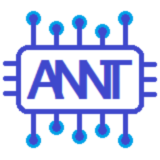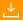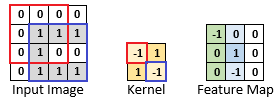# Conmajia

Stop stealing sheep!

## 卷积神经网络和图像分类识别

Andrew Kirillov 著
Conmajia 译
2019 年 1 月 15 日源码 491 KB

# 理论背景

1980 年，日本的福岛邦彦提出了种层次化的神经网络模型，命名为新认知机（neocongnitron）. 这个模型受简单和复杂细胞的概念的启发，新认知者能够通过学习物体的形状来识别模式.

## 卷积网络的架构

### 卷积层

$\tag{1} \mathbf{K}*\mathbf{I}=\sum_{i=1}^{n}\sum_{j=1}^{m}K_{n-i+1,m-j+1}*I_{i,j}$

$\begin{bmatrix} a & b & c \\ d & e & f \\ g & h & i \end{bmatrix} * \begin{bmatrix} 1 & 2 & 3 \\ 4 & 5 & 6 \\ 7 & 8 & 9 \end{bmatrix} \\ = i*1+h*2+g*3+f*4+e*5+d*6+c*7+b*8+a*9$

$$(1)$$ 的卷积定义是从信号处理领域借鉴过来的，核经过了垂直和水平翻转. 更直接的计算方法是 $$\mathbf{K}$$$$\mathbf{I}$$ 不进行翻转，直接进行正常点积. 这种操作称为互相关，定义如下：

$\mathbf{K}*\mathbf{I}=\sum_{i=1}^{n}\sum_{j=1}^{m}K_{i,j}*I_{i,j}$

$\tag{2} K^{(f)}*I_{y,x}=\sum_{l=1}^{d}\sum_{i=1}^{n}\sum_{j=1}^{m}\left[K_{l,i,j}^{(f)}*I_{l,y+i-1,x+j-1}+b^{(f)}\right],f=1,2,\cdots,z$

• $$w$$：输入特征图的宽度
• $$h$$：输入特征图的高度
• $$d$$：输入深度（特征图的数量）
• $$m$$：卷积核宽度
• $$n$$：卷积核高度
• $$z$$：卷积核数量（输出特征图的数量）

### ReLU 激活函数

ReLU 激活函数也就是 rectifier 激活函数，对卷积神经网络来说，它不是什么新东西. 随着更深层次的神经网络的兴起，它得到了广泛的推广.

ReLU 函数的定义为 $$f(x)=x^+=max(0,x)$$. 它最大的优点是，对于 $$x>0$$ 的值，它的导数总是 1，所以它允许更好的梯度传播，从而加快深度人工神经网络的训练速度. 和 sigmoid 和 $$\tanh$$ 相比，它的计算效率更高，速度更快.

### 建立卷积神经网络

LeNet-5 为例. 这是 Yann LeCun 提出的卷积神经网络结构，并应用于手写数字分类. 它输入 32×32 的灰度图像，产生 10 个值的向量，这些值代表数字属于某一类（数字 0 到 9）的概率. 表 2 总结了网络的结构、输出的尺寸和可训练参数（权重+偏差）的数量.

## 训练卷积网络

$\delta_i^{(\mathrm{net})}=\frac{\partial\mathrm{Cost}}{\partial\mathrm{net}_i}$

$\delta_i^{(k)}=\frac{\partial\mathrm{out}^{(k)}}{\partial\mathrm{in}^{(k)}_i}\delta^{(k+1)}$

$\delta_i^{(\mathrm{net})}=y_i-t_i$

$\delta_i^{(k)}=o_i(1-o_i)\delta_i^{(k+1)}$

$\delta_i^{(k)}=o_i(1-o_i^2)\delta_i^{(k+1)}$

$\delta_i^{(k)}=\sum_{j=1}^{m}\omega_{i,j}*\delta_j^{(k+1)}$

\begin{align*} \omega_{i,j}(t+1) &= \omega_{i,j}(t)-\lambda\left(\delta_i^{(k+1)}x_j\right) \\ b_i(t+1) &=b_i(t)-\lambda\delta_i^{(k+1)} \end{align*}

### 交叉熵成本函数

$\mathrm{Cost}=-\sum_{i=1}^{n}t_i\log(y_i)$

$\delta_i^{(\mathrm{net})}=-\frac{t_i}{y_i}$

《前馈》中已经介绍过在分类问题中用到的神经网络最后一层使用 sigmoid 作为激活函数. 它的输出值域为 $$(0,1)$$，可以理解为从 0% 到 100% 表示的概率. 如果神经网络输出层采用 sigmoid，它的确可能得到接近于真实值的概率. 但是现在要处理的是互斥类，很多情况下 sigmoid 的输出是无意义的. 比如上面的 4 类分类例子：一个输出向量是 {0.6,0.55,0.1,0.1}，这是用 sigmoid 可能得到的结果. 问题在哪？乍一看，这表明应该是第 1 类（60% 概率），但是第 2 类的可能性也很大（55%）. 而且这个输出结果有一个很大的问题，它的各概率和达到了 1.35，也就是目标属于这 4 类之一的可能性是 135%. 这在物理上是毫无意义的！ 这里要指出两个问题：第一，各分类概率和应为 100%，不能多，也不能少. 第二，对于难以识别的分类目标，如果目标既像第 1 类，又像第 2 类，那么怎么能确定 60% 这么高的概率一定是可信的？ 为了解决这两个问题，需要用到另一个激活函数：SoftMax. SoftMax 类似 sigmoid，值域也是 $$(0,1)$$. 不同的是，它处理整个输入向量而不是其中的单个值，这就保证了输出向量（概率）的和恒为 1. SoftMax 定义为： $f(x_i)=\frac{e^{x_i}}{\sum_{j=1}^{m}e^{x_j}}$ 将上面的例子改用 SoftMax 进行处理后，输出向量变得更合理了：$$\{0.316,0.3,0.192,0.192\}$$. 可以看到，向量中各概率的和等于 1，也就是 100%. 最可能的第 1 类，它的概率也不再高得离谱，只有 31.6%. 和其他激活函数一样，SoftMax 也需要定义它的梯度反向传播方程： \delta_i^{(k)}=\sum_{j=1}^{m}\left(\delta_j^{(k+1)}*\left\{ \begin{align*}j &=i,o_i(1-o_j) \\ j &\neq i,-o_io_j \end{align*} \right\}\right) 表 2 里可以看到 LeNet-5 神经网络架构中包含了全连接层和 sigmoid 激活函数. 这两者的方程也定义完毕，现在就可以继续讨论其他构建块了. ### ReLU 激活函数 前面提到过，ReLU 激活函数在深度神经网络经常用到，它对于大于 0 的输入向量梯度恒为 1，所以能保证误差梯度在网络中更好地传播. 现在来定义它的梯度反向传播方程： \delta_i^{(k)}=\delta_j^{(k+1)}*\left\{ \begin{align*} 1 &, o_i>0 \\ 0 &, o_i\leqslant0 \end{align*} \right\} ### 池化层 为了尽量简洁地说明误差梯度如何通过池化层反向传播，假设使用的池化层卷积核大小 2×2，跨度 2，不填充输入（只池化有效位置）. 这个假设意味着每个输出特征图的值都是基于 4 个值计算得到的. 尽管池化层假设输入向量是二维数据，但是下面的数学定义也可以处理输入输出是一维向量的情况. 首先定义 $$\mathrm{i2j}(i)$$ 函数，这个函数接受输入向量第 $$i$$ 个值（作为索引），并返回输出向量对应的第 $$j$$ 个值（作为索引）. 由于每个输出都是用 4 个输入值计算出来的，所以这意味着有 4 个 $$i$$ 会让 $$\mathrm{i2j}(i)$$ 函数输出同一个 $$j$$. 先从最大池化开始，定义误差梯度反向传播方程之前，还有一件事要做. 在正向传递时，计算神经网络的输出也会用与输出向量长度相同的最大索引值（max indices）向量填充池化层. 如果输出向量包含对应输入值的最大值，则最大索引值向量包含最大值的索引. 综上所述，可以定义最大池化层的梯度反向传播方程： \delta_i^{(k)}=\delta_{\mathrm{i2j}(i)}^{(k+1)}*\left\{ \begin{align*}1 &,i =p_{\mathrm{i2j}(i)} \\ 0 &, i\neq p_{\mathrm{i2j}(i)} \end{align*} \right\} 其中，$$p$$ 是最大索引值向量. 平均池化来说，就更简单了： $\delta_i^{(k)}=\frac{\delta_{\mathrm{i2j}(i)}^{(k+1)}}{q}$ 其中，$$q$$ 是卷积核大小，在这个例子里，$$q=4$$. ### 卷积层 最后来定义卷积层的反向传播过程. 牢记一点，它和全连接层的区别就在于共享权重和偏差值. 从卷积层的权重计算开始. 对于全连接层，误差对权重 $$\omega_{i,j}$$ 的偏导数等于下一个块的误差梯度乘以相应的输入值 $$\delta_i^{(k+1)}x_j$$. 这是因为每个输入／输出连接都在全连接层中分配了自己的权重，而全连接层是不共享的. 但是卷积层和这不一样，图 4 显示了卷积核的每个权重都用于多个输入／输出连接. 图中的例子，突出显示的卷积核权重每个使用了 9 次，对应输入图像中的 9 个不同位置. 因此，与权重有关的误差的偏导数也需要有 9 个.图 4 卷积层计算 和处理池化层时类似，这里忽略了卷积层处理的是二维／三维数据这一事实，而假设它们是普通的向量／数组（就像 C++ 编程时用到的那样）. 对于上面的示例，第一个权重（红线框出）应用于输入 $$\{1,2,3,5,6,7,9,10,11,13,14,15\}$$，而第四个权重应用于输入 $$\{6,7,8,10,11,12,14,15,16\}$$. 用 $$r_i$$ 表示每个权重使用的输入索引向量. 另外定义 $$\mathrm{i2o}(i,j)$$ 函数，它为第 $$i$$ 个权重和第 $$j$$ 个输入提供输出值索引. 上图中有几个例子，$$\mathrm{i2o}(1,1)=1$$$$\mathrm{i2o}(4,6)=1$$$$\mathrm{i2o}(1,11)=9$$$$\mathrm{i2o}(4,16)=9$$. 根据这些约定，可以定义卷积网络的权重： $\tag{3} \omega_i(t+1)=\omega_i(t)-\lambda\sum_{j}^{j\in r_i}\delta_{\mathrm{i2o}(i,j)}^{(k+1)}x_j$ 上面的玩意儿有什么意义？嗯，它的意义很丰富. 你想得越多，意义就越多. 这里的目标是为所有输出取误差梯度（因为每个核的权重用于计算所有的输出），然后将它们乘以相应的输入. 尽管有多个核，但是它们都以相同的模式应用，所以即使需要计算不同核的权重，权重输入向量也保持不变. 然而，$$\mathrm{i2o}(i,j)$$ 是每个核特定的，它可以使用核的索引作为额外的参数进行扩展. 更新偏差值要简单得多. 由于每个核／偏差都用于计算输出值，所以只需为当前核生成的特性图的误差梯度求和即可： $\tag{4} b(t+1)=b(t)-\lambda\sum_{j}^{s}\delta_j^{(k+1)}$ 其中，$$s$$ 是特性图. 式(3)$、式$(4)\$ 都是依据特征图／卷积核来完成的，权重和偏差值没有用核索引参数化.

$\delta_i^{(k)}=\sum_{j}^{j\in\gamma_i}\omega_{\mathrm{i2w}(i,j)}*\delta_j^{(k+1)}$

# 使用例程

## MNIST 手写数字分类

Conv(32x32x1, 5x5x6  ) -> ReLU -> AvgPool(2x2)
Conv(14x14x6, 5x5x16 ) -> ReLU -> AvgPool(2x2)
Conv(5x5x16,  5x5x120) -> ReLU
FC(120, 10) -> SoftMax


// 连接表用于指定第一卷积层要使用的由第二层生成的特征图
vector<bool> connectionTable( {
true,  true,  true,  false, false, false,
false, true,  true,  true,  false, false,
false, false, true,  true,  true,  false,
false, false, false, true,  true,  true,
true,  false, false, false, true,  true,
true,  true,  false, false, false, true,
true,  true,  true,  true,  false, false,
false, true,  true,  true,  true,  false,
false, false, true,  true,  true,  true,
true,  false, false, true,  true,  true,
true,  true,  false, false, true,  true,
true,  true,  true,  false, false, true,
true,  true,  false, true,  true,  false,
false, true,  true,  false, true,  true,
true,  false, true,  true,  false, true,
true,  true,  true,  true,  true,  true
} );

// 准备卷积神经网络
shared_ptr<XNeuralNetwork> net = make_shared<XNeuralNetwork>( );

net->AddLayer( make_shared<XConvolutionLayer>( 32, 32, 1, 5, 5, 6 ) );
net->AddLayer( make_shared<XAveragePooling>( 28, 28, 6, 2 ) );

net->AddLayer( make_shared<XConvolutionLayer>( 14, 14, 6, 5, 5, 16, connectionTable ) );
net->AddLayer( make_shared<XAveragePooling>( 10, 10, 16, 2 ) );

net->AddLayer( make_shared<XConvolutionLayer>( 5, 5, 16, 5, 5, 120 ) );

net->AddLayer( make_shared<XFullyConnectedLayer>( 120, 10 ) );


// 生成训练内容，用到了 Adam 优化器和负对数似然函数（SoftMax）
shared_ptr<XNetworkTraining> netTraining = make_shared<XNetworkTraining>( net,
make_shared<XNegativeLogLikelihoodCost>( ) );

// 使用助手类训练神经网络分类
XClassificationTrainingHelper trainingHelper( netTraining, argc, argv );
trainingHelper.SetValidationSamples( validationImages, encodedValidationLabels, validationLabels );
trainingHelper.SetTestSamples( testImages, encodedTestLabels, testLabels );

// 20 世代, 每批 50 样本
trainingHelper.RunTraining( 20, 50, trainImages, encodedTrainLabels, trainLabels );


MNIST handwritten digits classification example with Convolution ANN

Samples usage: training = 50000, validation = 10000, test = 10000

Learning rate: 0.0020, Epochs: 20, Batch Size: 50

Before training: accuracy = 5.00% (2500/50000), cost = 2.3175, 34.324s

Epoch   1 : [==================================================] 123.060s
Training accuracy = 97.07% (48536/50000), cost = 0.0878, 32.930s
Validation accuracy = 97.49% (9749/10000), cost = 0.0799, 6.825s
Epoch   2 : [==================================================] 145.140s
Training accuracy = 97.87% (48935/50000), cost = 0.0657, 36.821s
Validation accuracy = 97.94% (9794/10000), cost = 0.0669, 5.939s
...
Epoch  19 : [==================================================] 101.305s
Training accuracy = 99.75% (49877/50000), cost = 0.0077, 26.094s
Validation accuracy = 98.96% (9896/10000), cost = 0.0684, 6.345s
Epoch  20 : [==================================================] 104.519s
Training accuracy = 99.73% (49865/50000), cost = 0.0107, 28.545s
Validation accuracy = 99.02% (9902/10000), cost = 0.0718, 7.885s

Test accuracy = 99.01% (9901/10000), cost = 0.0542, 5.910s

Total time taken : 3187s (53.12min)


## CIFAR10 图片分类

Conv(32x32x3,  5x5x32, BorderMode::Same) -> ReLU -> MaxPool -> BatchNorm
Conv(16x16x32, 5x5x32, BorderMode::Same) -> ReLU -> MaxPool -> BatchNorm
Conv(8x8x32,   5x5x64, BorderMode::Same) -> ReLU -> MaxPool -> BatchNorm
FC(1024, 64) -> ReLU -> BatchNorm
FC(64, 10) -> SoftMax


ReLU(MaxPool)MaxPool(ReLU) 计算结果相同，但计算量减少 75%，所以这里选用前者.

// 准备卷积神经网络
shared_ptr<XNeuralNetwork> net = make_shared<XNeuralNetwork>( );

net->AddLayer( make_shared<XConvolutionLayer>( 32, 32, 3, 5, 5, 32, BorderMode::Same ) );
net->AddLayer( make_shared<XMaxPooling>( 32, 32, 32, 2 ) );
net->AddLayer( make_shared<XBatchNormalization>( 16, 16, 32 ) );

net->AddLayer( make_shared<XConvolutionLayer>( 16, 16, 32, 5, 5, 32, BorderMode::Same ) );
net->AddLayer( make_shared<XMaxPooling>( 16, 16, 32, 2 ) );
net->AddLayer( make_shared<XBatchNormalization>( 8, 8, 32 ) );

net->AddLayer( make_shared<XConvolutionLayer>( 8, 8, 32, 5, 5, 64, BorderMode::Same ) );
net->AddLayer( make_shared<XMaxPooling>( 8, 8, 64, 2 ) );
net->AddLayer( make_shared<XBatchNormalization>( 4, 4, 64 ) );

net->AddLayer( make_shared<XFullyConnectedLayer>( 4 * 4 * 64, 64 ) );
net->AddLayer( make_shared<XBatchNormalization>( 64, 1, 1 ) );

net->AddLayer( make_shared<XFullyConnectedLayer>( 64, 10 ) );


CIFAR-10 dataset classification example with Convolutional ANN

Samples usage: training = 43750, validation = 6250, test = 10000

Learning rate: 0.0010, Epochs: 20, Batch Size: 50

Before training: accuracy = 9.91% (4336/43750), cost = 2.3293, 844.825s

Epoch   1 : [==================================================] 1725.516s
Training accuracy = 48.25% (21110/43750), cost = 1.9622, 543.087s
Validation accuracy = 47.46% (2966/6250), cost = 2.0036, 77.284s
Epoch   2 : [==================================================] 1742.268s
Training accuracy = 54.38% (23793/43750), cost = 1.3972, 568.358s
Validation accuracy = 52.93% (3308/6250), cost = 1.4675, 76.287s
...
Epoch  19 : [==================================================] 1642.750s
Training accuracy = 90.34% (39522/43750), cost = 0.2750, 599.431s
Validation accuracy = 69.07% (4317/6250), cost = 1.2472, 81.053s
Epoch  20 : [==================================================] 1708.940s
Training accuracy = 91.27% (39931/43750), cost = 0.2484, 578.551s
Validation accuracy = 69.15% (4322/6250), cost = 1.2735, 81.037s

Test accuracy = 68.34% (6834/10000), cost = 1.3218, 122.455s

Total time taken : 48304s (805.07min)


# 关于作者Andrew Kirillov，来自英国🇬🇧，目前就职于 IBM.

1. Yann LeCun，中文名杨立昆，Facebook 首席人工智能专家，人工智能研究院院长，被称为“深度学习三大巨头”之一，另外两位是 Geoffrey Hinton 和 Yoshua Bengio. ↩︎

posted on 2019-01-16 22:41  Conmajia  阅读(9343)  评论(0编辑  收藏  举报